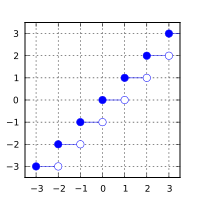/C

# floor, floorf, floorl

Defined in header `<math.h>`
`float       floorf( float arg );`
(1) (since C99)
`double      floor( double arg );`
(2)
`long double floorl( long double arg );`
(3) (since C99)
Defined in header `<tgmath.h>`
`#define floor( arg )`
(4) (since C99)
1-3) Computes the largest integer value not greater than `arg`.
4) Type-generic macro: If `arg` has type `long double`, `floorl` is called. Otherwise, if `arg` has integer type or the type `double`, `floor` is called. Otherwise, `floorf` is called.

### Parameters

 arg - floating point value

### Return value

If no errors occur, the largest integer value not greater than `arg`, that is ⌊arg⌋, is returned.

Return valueArgument

### Error handling

Errors are reported as specified in math_errhandling.

If the implementation supports IEEE floating-point arithmetic (IEC 60559),

• The current rounding mode has no effect.
• If `arg` is ±∞, it is returned, unmodified
• If `arg` is ±0, it is returned, unmodified
• If arg is NaN, NaN is returned

`FE_INEXACT` may be (but isn't required to be) raised when rounding a non-integer finite value.

The largest representable floating-point values are exact integers in all standard floating-point formats, so this function never overflows on its own; however the result may overflow any integer type (including `intmax_t`), when stored in an integer variable.

### Example

```#include <math.h>
#include <stdio.h>
int main(void)
{
printf("floor(+2.7) = %+.1f\n", floor(2.7));
printf("floor(-2.7) = %+.1f\n", floor(-2.7));
printf("floor(-0.0) = %+.1f\n", floor(-0.0));
printf("floor(-Inf) = %+f\n",   floor(-INFINITY));
}```

Possible output:

```floor(+2.7) = +2.0
floor(-2.7) = -3.0
floor(-0.0) = -0.0
floor(-Inf) = -inf```
• C11 standard (ISO/IEC 9899:2011):
• 7.12.9.2 The floor functions (p: 251)
• 7.25 Type-generic math <tgmath.h> (p: 373-375)
• F.10.6.2 The floor functions (p: 526)
• C99 standard (ISO/IEC 9899:1999):
• 7.12.9.2 The floor functions (p: 232)
• 7.22 Type-generic math <tgmath.h> (p: 335-337)
• F.9.6.2 The floor functions (p: 463)
• C89/C90 standard (ISO/IEC 9899:1990):
• 4.5.6.3 The floor function

### See also

 ceilceilfceill (C99)(C99) computes smallest integer not less than the given value (function) trunctruncftruncl (C99)(C99)(C99) rounds to nearest integer not greater in magnitude than the given value (function) roundlroundllround (C99)(C99)(C99) rounds to nearest integer, rounding away from zero in halfway cases (function)

© cppreference.com
Licensed under the Creative Commons Attribution-ShareAlike Unported License v3.0.
http://en.cppreference.com/w/c/numeric/math/floor